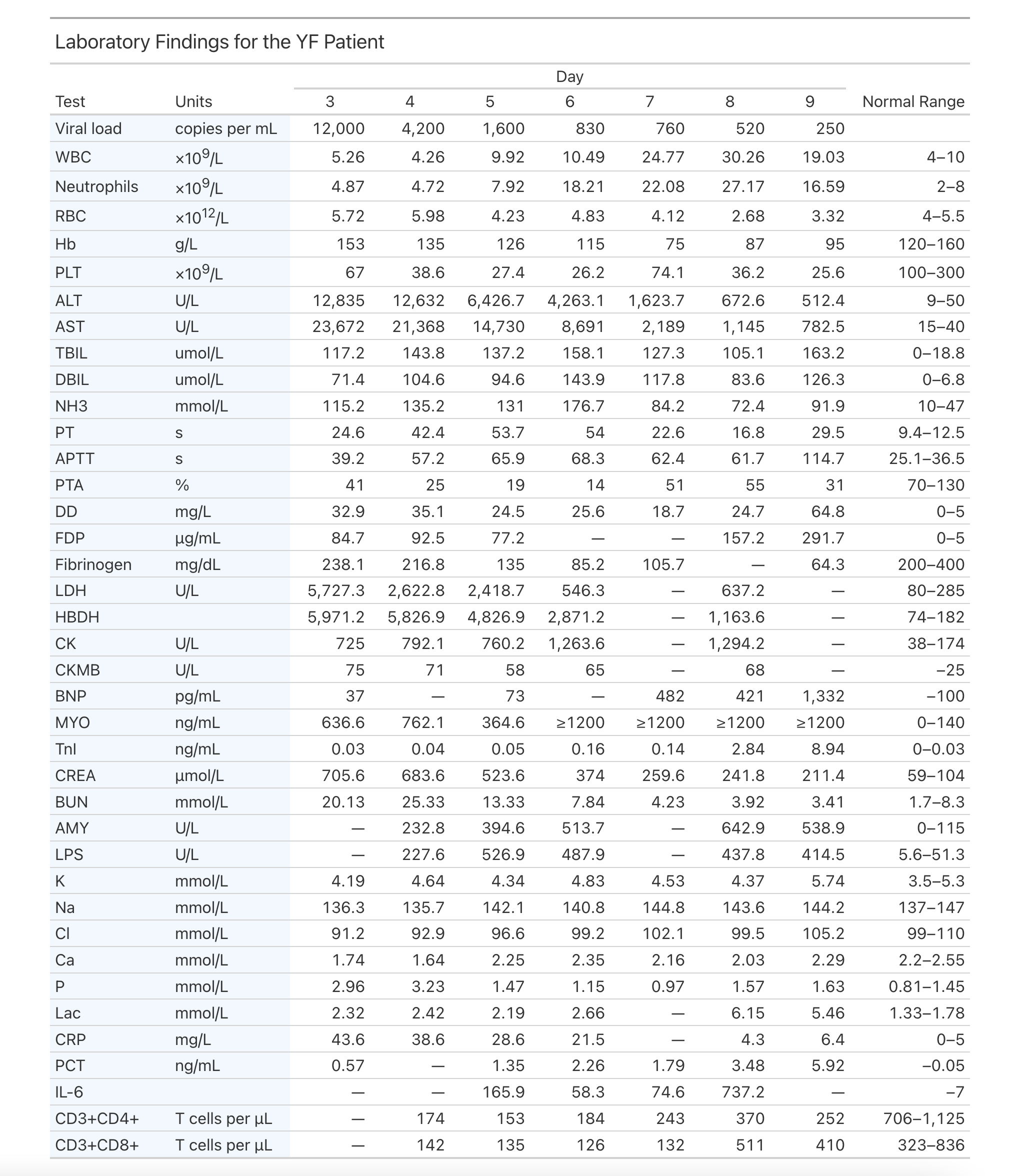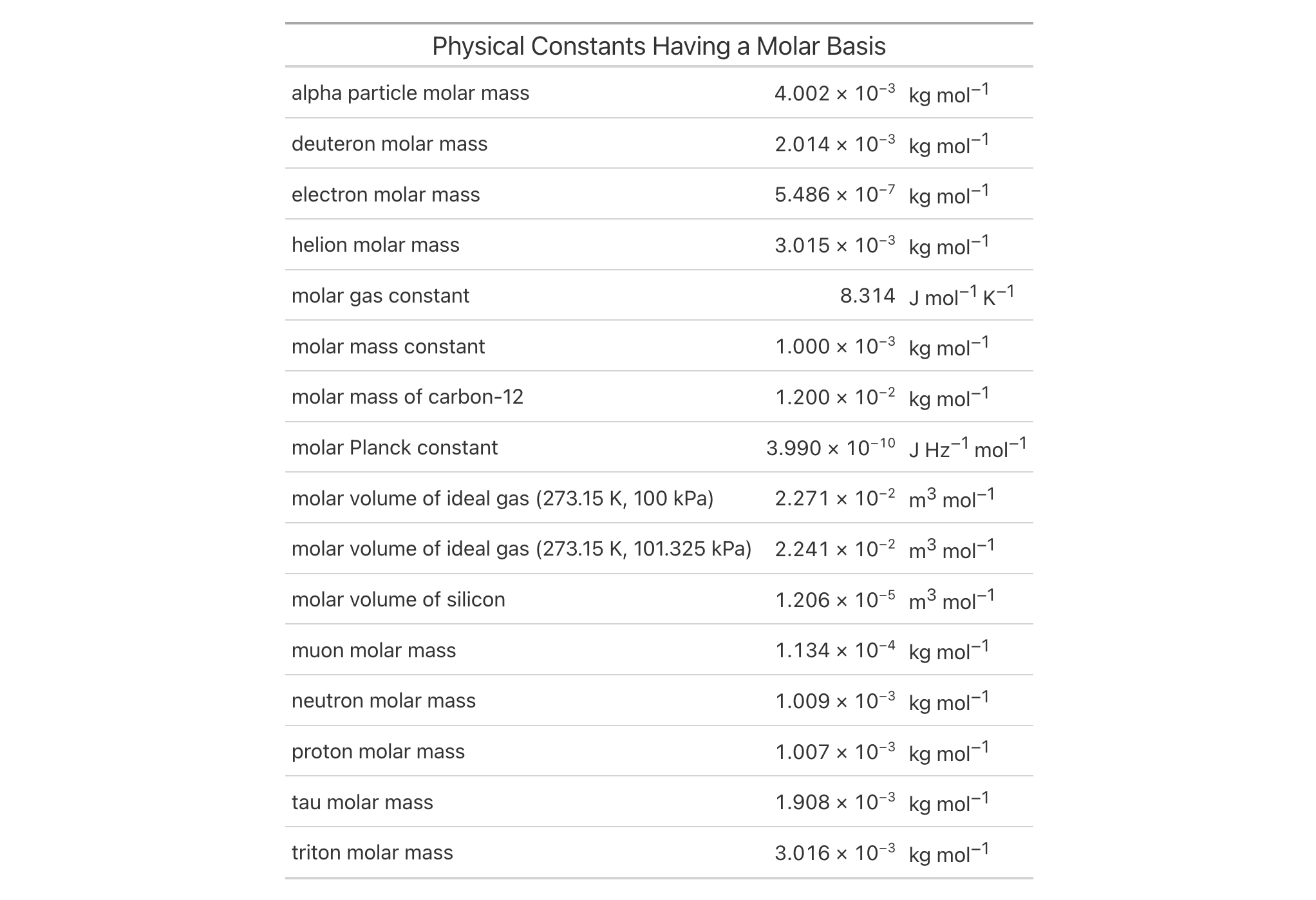The fmt_units() function lets you better format measurement units in the table body. These must conform to gt's specialized units notation (e.g., "J Hz^-1 mol^-1" can be used to generate units for the molar Planck constant) for the best conversion. The notation here provides several conveniences for defining units, so as long as the values to be formatted conform to this syntax, you'll obtain nicely-formatted units no matter what the table output format might be (i.e., HTML, LaTeX, RTF, etc.). Details pertaining to the units notation can be found in the section entitled How to use gt's units notation.

## Usage

fmt_units(data, columns = everything(), rows = everything())

## Arguments

data

The gt table data object

obj:<gt_tbl> // required

This is the gt table object that is commonly created through use of the gt() function.

columns

Columns to target

<column-targeting expression> // default: everything()

Can either be a series of column names provided in c(), a vector of column indices, or a select helper function. Examples of select helper functions include starts_with(), ends_with(), contains(), matches(), one_of(), num_range(), and everything().

rows

Rows to target

<row-targeting expression> // default: everything()

In conjunction with columns, we can specify which of their rows should undergo formatting. The default everything() results in all rows in columns being formatted. Alternatively, we can supply a vector of row captions within c(), a vector of row indices, or a select helper function. Examples of select helper functions include starts_with(), ends_with(), contains(), matches(), one_of(), num_range(), and everything(). We can also use expressions to filter down to the rows we need (e.g., [colname_1] > 100 & [colname_2] < 50).

## Value

An object of class gt_tbl.

## Targeting cells with columns and rows

Targeting of values is done through columns and additionally by rows (if nothing is provided for rows then entire columns are selected). The columns argument allows us to target a subset of cells contained in the resolved columns. We say resolved because aside from declaring column names in c() (with bare column names or names in quotes) we can use tidyselect-style expressions. This can be as basic as supplying a select helper like starts_with(), or, providing a more complex incantation like

where(~ is.numeric(.x) && max(.x, na.rm = TRUE) > 1E6)

which targets numeric columns that have a maximum value greater than 1,000,000 (excluding any NAs from consideration).

By default all columns and rows are selected (with the everything() defaults). Cell values that are incompatible with a given formatting function will be skipped over, like character values and numeric fmt_*() functions. So it's safe to select all columns with a particular formatting function (only those values that can be formatted will be formatted), but, you may not want that. One strategy is to format the bulk of cell values with one formatting function and then constrain the columns for later passes with other types of formatting (the last formatting done to a cell is what you get in the final output).

Once the columns are targeted, we may also target the rows within those columns. This can be done in a variety of ways. If a stub is present, then we potentially have row identifiers. Those can be used much like column names in the columns-targeting scenario. We can use simpler tidyselect-style expressions (the select helpers should work well here) and we can use quoted row identifiers in c(). It's also possible to use row indices (e.g., c(3, 5, 6)) though these index values must correspond to the row numbers of the input data (the indices won't necessarily match those of rearranged rows if row groups are present). One more type of expression is possible, an expression that takes column values (can involve any of the available columns in the table) and returns a logical vector. This is nice if you want to base formatting on values in the column or another column, or, you'd like to use a more complex predicate expression.

## How to use gt's units notation

The units notation involves a shorthand of writing units that feels familiar and is fine-tuned for the task at hand. Each unit is treated as a separate entity (parentheses and other symbols included) and the addition of subscript text and exponents is flexible and relatively easy to formulate. This is all best shown with examples:

• "m/s" and "m / s" both render as "m/s"

• "m s^-1" will appear with the "-1" exponent intact

• "m \s" gives the the same result, as "\<unit>" is equivalent to "<unit>^-1"

• "E_h" will render an "E" with the "h" subscript

• "t_i^2.5" provides a t with an "i" subscript and a "2.5" exponent

• "m[_0^2]" will use overstriking to set both scripts vertically

• "g/L %C6H12O6%" uses a chemical formula (enclosed in a pair of "%" characters) as a unit partial, and the formula will render correctly with subscripted numbers

• Common units that are difficult to write using ASCII text may be implicitly converted to the correct characters (e.g., the "u" in "ug", "um", "uL", and "umol" will be converted to the Greek mu symbol; "degC" and "degF" will render a degree sign before the temperature unit)

• We can transform shorthand symbol/unit names enclosed in ":" (e.g., ":angstrom:", ":ohm:", etc.) into proper symbols

• The components of a unit (unit name, subscript, and exponent) can be fully or partially italicized/emboldened by surrounding text with "*" or "**"

## Examples

Let's use the illness dataset and create a new gt table. The units column contains character values in gt's specialized units notation (e.g., "x10^9 / L") so the fmt_units() function was used to better format those units.

illness |>
gt() |>
fmt_units(columns = units) |>
sub_missing(columns = -starts_with("norm")) |>
sub_missing(columns = c(starts_with("norm"), units), missing_text = "") |>
sub_large_vals(rows = test == "MYO", threshold = 1200) |>
fmt_number(
decimals = 2,
drop_trailing_zeros = TRUE
) |>
tab_header(title = "Laboratory Findings for the YF Patient") |>
tab_spanner(label = "Day", columns = starts_with("day")) |>
cols_label_with(fn = ~ gsub("day_", "", .)) |>
cols_merge_range(col_begin = norm_l, col_end = norm_u) |>
cols_label(
starts_with("norm") ~ "Normal Range",
test ~ "Test",
units ~ "Units"
) |>
cols_width(
starts_with("day") ~ px(80),
everything() ~ px(120)
) |>
tab_style(
style = cell_text(align = "center"),
locations = cells_column_labels(columns = starts_with("day"))
) |>
tab_style(
style = cell_fill(color = "aliceblue"),
locations = cells_body(columns = c(test, units))
) |>
tab_options(heading.padding = px(10))The constants dataset contains values for hundreds of fundamental physical constants. We'll take a subset of values that have some molar basis and generate a gt table from that. Like the illness dataset, this one has a units column so, again, the fmt_units() function will be used to format those units. Here, the preference for typesetting measurement units is to have positive and negative exponents (e.g., not "<unit_1> / <unit_2>" but rather "<unit_1> <unit_2>^-1").

constants |>
dplyr::filter(grepl("molar", name)) |>
gt() |>
cols_hide(columns = c(uncert, starts_with("sf"))) |>
fmt_units(columns = units) |>
fmt_scientific(columns = value, decimals = 3) |>
tab_header(title = "Physical Constants Having a Molar Basis") |>
tab_options(column_labels.hidden = TRUE)3-18

## Function Introduced

In Development

Other data formatting functions: data_color(), fmt_auto(), fmt_bins(), fmt_bytes(), fmt_currency(), fmt_datetime(), fmt_date(), fmt_duration(), fmt_engineering(), fmt_flag(), fmt_fraction(), fmt_icon(), fmt_image(), fmt_index(), fmt_integer(), fmt_markdown(), fmt_number(), fmt_partsper(), fmt_passthrough(), fmt_percent(), fmt_roman(), fmt_scientific(), fmt_spelled_num(), fmt_time(), fmt_url(), fmt(), sub_large_vals(), sub_missing(), sub_small_vals(), sub_values(), sub_zero()